# Straight-line depreciation

Straight-line depreciation (SL) is a method used to perform depreciation expense recognition. Depreciation expense recognition ensures that the cost of long-lived assets is matched with revenues. Long-lived assets, i.e. assets that are used for more than one accounting period, provide economic benefits that are spread over time. In the case tangible assets, we call the allocation of cost over an asset’s life depreciation. In the case of intangible assets, we call it amortization. For natural resources, we use the term depletion.

On this page, we discuss the 3 methods of depreciation, how to depreciate an asset, and include a straight line depreciation calculator and a double declining balance depreciation calculator.

## 3 methods of depreciation

Generally speaking, there are 3 methods of depreciation: (1) straight-line method of depreciation, declining balance method of depreciation, and (3) units-of-production method.

## Straight-line method of depreciation

The straight-line method of depreciation or linear depreciation method recognizes an equal amount of depreciation expense each year. As a consequence, the carrying value of the assets goes down linearly to the salvage value. As such, a straight line depreciation schedule is very simple, making the SL the simplest method of depreciation. Unfortunately, it does not consider the fact that most assets lose most of their carrying value in the early years. To take this aspect into account, we should use an accelerated depreciation method.

## Declining balance method of depreciation

The declining balancing method (DB) applies a constant rate of depreciation to an asset’s carrying value each year. The most common declining balance method is the double-declining balance (DDB) method. This method applies two times the straight line rate to the declining balance.

## Units-of-production method

The first two methods of calculating depreciation do not consider the actual use of the assets, whereas the units-of-production does. In particular, the units-of-production method bases depreciation on usage. Thus, depreciation expense will be higher in periods of higher usage.

## Straight-line depreciation formula

How to calculate straight line depreciation? Of these three forms of depreciation, the straight-line depreciation is actually the simplest one. The formula to determine the annual depreciation expense is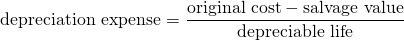We implement a straight line method depreciation calculator at the bottom of this page using exactly this formula.

## Double declining depreciation formula

In the case of double-declining depreciation method, the depreciation expense does not depend on the salvage value. However, once the book value of the asset reaches the salvage value, we no longer depreciate the asset.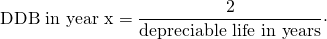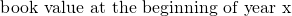Also in the Excel spreadsheet below, we implement the diminishing value depreciation calculator. This declining balance depreciation calculator allows us to look at the impact of changing the rate of depreciation on annual depreciation.

## Units-of-production depreciation formula

Finally, the formula for the units-of-production depreciation equals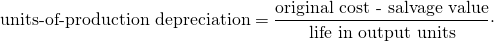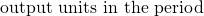## Component depreciation

IFRS requires that components of an asset are depreciated separately. To do this, we need useful life estimates for every component. It is also allowed under US GAAP but not used often.

## Amortization vs depreciation

As we mentioned in the introduction, the difference between amortization vs depreciation is related to the nature of the assets. In the case of tangible assets, we refer to depreciation. In the case of intangible assets, we use amortization. The methods to calculate the annual expense, however, are exactly the same.

## Straight-line depreciation example

In the following figure, we plot the annual depreciation for a machine that costs $1,200, has a residual value of$200 and that has a useful life of 5 years.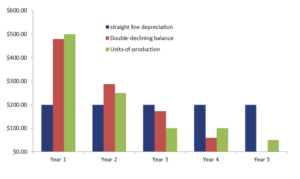## Summary

We discussed the methods of calculating depreciation. These three methods are straightforward and can easily be implemented in Excel. The spreadsheet below implements an example of each method.

### Straight-line depreciation calculator

Want to have an implementation in Excel? Download the Excel file: Straight-line Depreciation